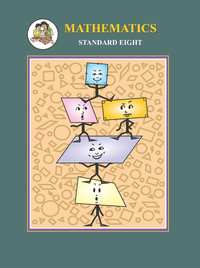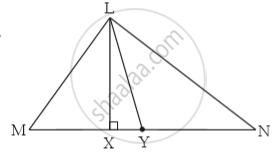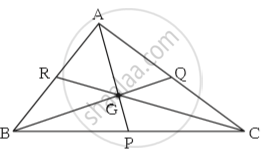Share

# Balbharati solutions for Maharashtra state board Class 8 Mathematics chapter 4 - Altitudes and Medians of a triangle [Latest edition]

Textbook page

#### Chapters## Chapter 4: Altitudes and Medians of a triangle

Practice Set 4.1

#### Balbharati solutions for Maharashtra state board Class 8 Mathematics Chapter 4 Altitudes and Medians of a triangle Exercise Practice Set 4.1 [Page 22]

Practice Set 4.1 | Q 1 | Page 22

In  LMN, _____ is an altitude and _____ is a median. (write the names of appropriate segments.)Practice Set 4.1 | Q 2 | Page 22

Draw an acute angled Δ PQR. Draw all of its altitudes. Name the point of concurrence as ‘O’.

Practice Set 4.1 | Q 3 | Page 22

Draw an obtuse angled Δ STV. Draw its medians and show the centroid.

Practice Set 4.1 | Q 4 | Page 22

Draw an obtuse angled Δ LMN. Draw its altitudes and denote the orthocentre by ‘O’.

Practice Set 4.1 | Q 5 | Page 22

Draw a right angled Δ XYZ. Draw its medians and show their point of concurrence by G.

Practice Set 4.1 | Q 6 | Page 22

Draw an isosceles triangle. Draw all of its medians and altitudes. Write your observation about their points of concurrence.

Practice Set 4.1 | Q 7 | Page 22

Fill in the blanks.Point G is the centroid of  ABC.
(1) If l(RG) = 2.5 then l(GC) = _____
(2) If l(BG) = 6 then l(BQ) = _____
(3) If l(AP) = 6 then l(AG) = _____   and l(GP) = _____

## Chapter 4: Altitudes and Medians of a triangle

Practice Set 4.1## Balbharati solutions for Maharashtra state board Class 8 Mathematics chapter 4 - Altitudes and Medians of a triangle

Balbharati solutions for Maharashtra state board Class 8 Mathematics chapter 4 (Altitudes and Medians of a triangle) include all questions with solution and detail explanation. This will clear students doubts about any question and improve application skills while preparing for board exams. The detailed, step-by-step solutions will help you understand the concepts better and clear your confusions, if any. Shaalaa.com has the Maharashtra State Board Maharashtra state board Class 8 Mathematics solutions in a manner that help students grasp basic concepts better and faster.

Further, we at Shaalaa.com provide such solutions so that students can prepare for written exams. Balbharati textbook solutions can be a core help for self-study and acts as a perfect self-help guidance for students.

Concepts covered in Maharashtra state board Class 8 Mathematics chapter 4 Altitudes and Medians of a triangle are Altitudes of a Triangle, Medians of a Triangle.

Using Balbharati Class 8 solutions Altitudes and Medians of a triangle exercise by students are an easy way to prepare for the exams, as they involve solutions arranged chapter-wise also page wise. The questions involved in Balbharati Solutions are important questions that can be asked in the final exam. Maximum students of Maharashtra State Board Class 8 prefer Balbharati Textbook Solutions to score more in exam.

Get the free view of chapter 4 Altitudes and Medians of a triangle Class 8 extra questions for Maharashtra state board Class 8 Mathematics and can use Shaalaa.com to keep it handy for your exam preparation